Thursday, August 5, 2021
Home > Latest Announcement > NMTC Final Stage Previous Year Question Papers For Class 7 and 8

# NMTC Final Stage Previous Year Question Papers For Class 7 and 8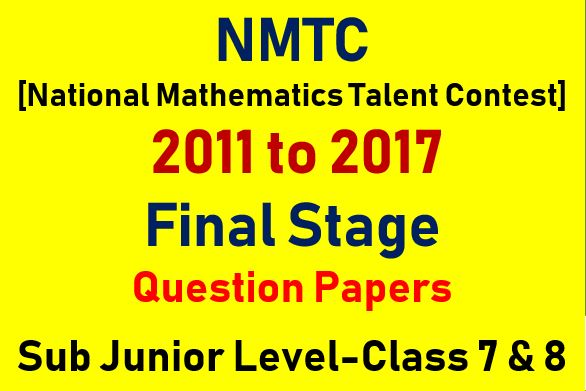Hi students, welcome to Amans Maths Blogs (AMB). Are you an aspirant of any competitive exams like CBSE Board, JEE, NTSE, NDA, NMTC etc.? If yes, then it is very important to solve the previous year questions papers to get the knowledge of the paper pattern of that particular examination. So, in this article, the students will get the NMTC final stage previous year question papers for class 7 and 8. These NMTC final stage previous year question papers are important for those students who qualify the NTSE Stage 1 exam.

Now, the question is why it is important to solve NMTC final stage previous year question papers for class 7 and 8.

This is because solving the NMTC stage 2 previous year question papers will help the aspirants of NMTC to get the blend knowledge and depth of the concepts and solve those tricky questions quickly.

NMTC final stage previous question paper will help the aspirants together a fair idea about the difficulty level of different sections of NMTC.

The aspirants must practice the NMTC final stage previous year question papers for class 7 and 8 in order to get the exams pattern of NMTC final stage.

These previous year question papers will help the candidate to learn about the trending topics and most of the frequently asked questions type in the exams.

Solving NMTC stage 2 previous year question papers also help the students to know their weak areas in the whole syllabus and work on them.

NMTC Final Stage Previous Year 2011 Question Papers For Class 7 and 8

Instructions:

1. Answer as many questions as possible.
2. Elegant and novel solutions will be get extra credits.
3. Diagram and explanations should be given wherever necessary.
4. Fill in FACE SLIP and your rough working should be in the answer book.
5. Maximum time allowed time is 3 hours.
6. All questions carry equal marks.

NMTC Final Stage Previous Year Question For Class 7 and 8: Ques No 1

Find all three-digit and four-digit natural numbers such that the product of the digits is a prime number. Find the sum of all such three-digit numbers and the sum of all such four digit numbers. Find the biggest prime factor of each sum.

NMTC Final Stage Previous Year Question For Class 7 and 8: Ques No 2

Let a sequence of numbers be denoted as t1, t2, t3, … where t1 = 1 and tn = t(n – 1) + n (n is a natural number). Find t2, t3, t4, t10, t2011.

NMTC Final Stage Previous Year Question For Class 7 and 8: Ques No 3

When written out completely 162011 has m digits and 6252011 has n digits. Find the value of (m + n).

NMTC Final Stage Previous Year Question For Class 7 and 8: Ques No 4

Four-digit numbers are formed by four different digits a, b, c, d (none of them is zero) without any repetition of digits. Prove that when the sum of all such numbers when divided by the sum of all such numbers when divided by the sum of the digits a, b, c, d the quotient is 6666.

NMTC Final Stage Previous Year Question For Class 7 and 8: Ques No 5

ABCD is a parallelogram. Through C is a straight line is drawn outside the parallelogram. AP, BQ, DR are drawn perpendicular to this line from A, B and D. Prove that AP = BQ + DR

NMTC Final Stage Previous Year Question For Class 7 and 8: Ques No 6

Neev puts 12 plastic bags inside another plastic bag. Each of these 12 bags is either empty or contains 12 other plastic bags. All together if 12 bags were non-empty, find the total number of bags.

NMTC Final Stage Previous Year Question For Class 7 and 8: Ques No 7

Nine squares are arranged to form a rectangle ABCD. The smallest square P has an area 4 sq. units. Find the areas of Q and R.NMTC Final Stage Previous Year 2012 Question Papers For Class 7 and 8

Instructions:

1. Same as Previous Instructions

NMTC Final Stage Previous Year Question For Class 7 and 8: Ques No 1

Find the number of numbers coprime to less than 2012. Find their sum. Find also the quotient when this sum is divided by 2012. (Information 503 is prime).

NMTC Final Stage Previous Year Question For Class 7 and 8: Ques No 2

Composite twins are defined as below.

(a) Odd composite twine: Let a and (a + 2) be two odd composite numbers. If (a – 2) and (a + 4) are primes then (a, a + 2) is called an odd composite twin.

(b) Even composite twine: Let b and (b + 2) are two even numbers (b > 2). If (b – 1) and (b + 3) are primes then (b, b + 2) is called an even composite twin.

List all composite twins less than or equal to 100.

NMTC Final Stage Previous Year Question For Class 7 and 8: Ques No 3

ABCD is a rectangle. The sides are extended and the external angles are bisected and the bisectors are produced in both ways to form a quadrilateral. Prove that the quadrilateral is a square.

NMTC Final Stage Previous Year Question For Class 7 and 8: Ques No 4

(a) A single digit natural number is increased by 10. The obtained number is now increased by the same percentage as in the first increase. The result is 72. Find the original single digit number.

(b) After two price reductions by one and the same percent the price of an article is reduced from Rs. 250 to Rs. 160. By how much percent was the price reduced each time. Write detailed steps.

NMTC Final Stage Previous Year Question For Class 7 and 8: Ques No 5

If a finite straight line segment is divided into two parts so that the rectangle contained by the whole and first part is equal to the square on the other part, prove that the square described on one of the diagonals of the rectangle contained by the whole and the first part is three times the square on the other part.

NMTC Final Stage Previous Year Question For Class 7 and 8: Ques No 6

abcde is a five-digit number. Show that abcde is divisible by 7 if and only if the number abcd – 2e is divisible by 7.

NMTC Final Stage Previous Year Question For Class 7 and 8: Ques No 7

If a2x3 + b2y3 + c2z3 = p5, ax2 = by2 = cz2 and 1/x + 1/y + 1/z = 1/p. Find the value of only in terms of p.

NMTC Final Stage Previous Year Question For Class 7 and 8: Ques No 8

Take any natural number. Multiply it with the next two natural numbers. Take another natural number different from the first and do the same as before. Subtract one result from the other to get a positive difference and divide the difference obtained by the positive difference of the original numbers. Add to the quotient the product of the original numbers. Prove that the final result if the product of some number by the number next above it.

NMTC Final Stage Previous Year 2014 Question Papers For Class 7 and 8

Instructions:

1. Same as Previous Instructions

NMTC Final Stage Previous Year Question For Class 7 and 8: Ques No 1

A hare pursued by a grey-hound is 50 of her own leaps ahead of him. While the hare takes 4 leaps the grey-hound takes 3 leaps. In one leaps the hare goes 1(3/4) meter and the grey-hound 2(3/4) meter. In how many leaps will the grey-hound overtake the hare?

NMTC Final Stage Previous Year Question For Class 7 and 8: Ques No 2

If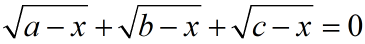, show that (a + b + c + 3x)(a + b + c – x) = 4(ab + bc + ca).

NMTC Final Stage Previous Year Question For Class 7 and 8: Ques No 3

Some amount of work has to be completed. Anand, Bilal and Charles offered to do the job. Anand would alone take a times as many days as Bilal and Chrles working together. Bilal would alone take b times as many day as many as Anand and Charles together. Charles would alone take c times as many days as Anand and Bilal together. Show that a/(a + 1) + b/(b + 1) + c/(c + 1) = 2.

NMTC Final Stage Previous Year Question For Class 7 and 8: Ques No 4

Let P be any point on the diagonal BD of a rectangle ABCD. F is the foot of the perpendicular fro P to BC. H is a point on the side BC such that FB = FH. PC cuts AH in Q. Show that the area of ΔAPQ = area of ΔCHQ.

NMTC Final Stage Previous Year Question For Class 7 and 8: Ques No 5

A number of three-digits in scale 7 when expressed in scale 9 has its digits reversed in order. Find the number in scale 7 and scale 10.

NMTC Final Stage Previous Year Question For Class 7 and 8: Ques No 6

(a)Two regular polygon have the number of their sides as 3:2 and the interior angles as 10:9. Find the number in sides of the polygons.

(b) Find the two natural numbers such that their difference, sum and the product is to one another as 1, 7 and 24.

NMTC Final Stage Previous Year Question For Class 7 and 8: Ques No 7

Find the value of a, b, c which will make each of the expressions x4 + ax3 + bx2 + cx + 1 and x4 + 2ax3 + 2bx2 + 2cx + 1 a perfect square.

NMTC Final Stage Previous Year 2015 Question Papers For Class 7 and 8

Instructions:

1. Same as Previous Instructions

NMTC Final Stage Previous Year Question For Class 7 and 8: Ques No 1

(a) The diagram below contains 13 boxes. The numbers in the second and the twelfth boxes are respectively 175 and 70.Fill up in the boxes with natural numbers such that (i) Sum of all numbers in all the 13 boxes is 2015 (ii) Sum of the numbers in any three consecutive boxes is always the same. The solution must contain the steps how you arrive at the numbers.

(b) If x, y, z are real and unequal numbers, prove that 2015x2 + 2015y2 + 6z2 > 2(2012xy + 3yz + 3zx).

NMTC Final Stage Previous Year Question For Class 7 and 8: Ques No 2

If a, b, c are reals such that a + b = 4 and 2c2 – ab = 4c – 10, find the numerical values of a, b and c.

NMTC Final Stage Previous Year Question For Class 7 and 8: Ques No 3

When a = 22014 and b = 22015,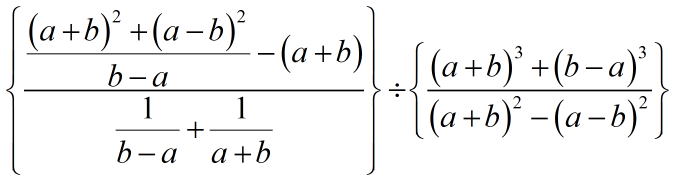prove that is divisible by 3

NMTC Final Stage Previous Year Question For Class 7 and 8: Ques No 4

Prove that the feet of the perpendicular drawn from the vertices of a parallelogram on to its diagonals are the vertices of a parallelogram.

NMTC Final Stage Previous Year Question For Class 7 and 8: Ques No 5

ABC is an acute angled triangle. PQ are the points on AB and AC respectively such that the area of ΔAPC = area of ΔAQB. A line is drawn through B is parallel to AC and meets the line through Q parallel to AB at S. QS cuts BC at R. Prove that RS = AP.

NMTC Final Stage Previous Year Question For Class 7 and 8: Ques No 6

(a) A man is walking from a town A to another town B at a speed of 4 km/hr. He started one hour before a bus starts. The bus is travelling with a speed of 12 km/he. The man on the way got into the bus and travels 2 hours and reached town B. What is the distance between town A and town B.

(b) A point P is taken within a rhombus ABCD such that PA = PC. Show that B, P, D are collinear.

NMTC Final Stage Previous Year Question For Class 7 and 8: Ques No 7

If (x + y + z)3 = (y + z – x)3 + (z + x – y)3 + (x + y – z)3 + kxyz. Find the numerical value of k. Deduce the following result. If a = 2015, b = 2014, c = 1/2014, then prove that (a + b + c)3 – (a + b – c)3 – (b + c – a)3 – (c + a – b)3 – 23abc = 2015.

NMTC Final Stage Previous Year 2016 Question Papers For Class 7 and 8

Instructions:

1. Same as Previous Instructions

NMTC Final Stage Previous Year Question For Class 7 and 8: Ques No 1

(a) If x/a = y/b = z/c = 2016, where x, y, z, a, b, c are non-zero real numbers, find the value of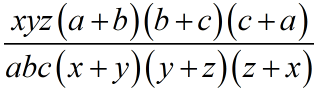.

(b) Four boysAmar, benny, Charan, Dany and four girls Azija, Beula, Chitra and Dais have to work on a project. They should from 4 pairs, one boy and one girl in each. They know each other with the following constraints:

(i) Amar knows neither Azija nor Buela,

(ii) Benny does not know Buela,

(iii) Both Charan and Deny know neither Chitra nor Daisy.

In how many ways can the pairs be formed so that each boy knows the girl in his pair.

NMTC Final Stage Previous Year Question For Class 7 and 8: Ques No 2

In a triangle ABC, ∠ C = 90° and BC = 3AC. Points D, E lie on CB such that CD = DE = EB. Prove that ∠ ABC + ∠ AEC + ∠ ADC = 90°.

NMTC Final Stage Previous Year Question For Class 7 and 8: Ques No 3

Let m, n, p be distinct two digit natural numbers. If m = 10a + b, n = 10b + c, p = 10c + a. Find all possible values of GCD(m, n, p).

NMTC Final Stage Previous Year Question For Class 7 and 8: Ques No 4

If xy = ab(a + b) and x2 + y2 – xy = a3 + b3, find the value of (x/a – y/b)(x/b – y/a).

NMTC Final Stage Previous Year Question For Class 7 and 8: Ques No 5

The square ABCD of side length a cm is rotated about A in the clockwise direction by an angle 45° to become the square AB’C’D’. Show that the shaded area is  square unit.NMTC Final Stage Previous Year 2017 Question Papers For Class 7 and 8

Instructions:

1. Same as Previous Instructions

NMTC Final Stage Previous Year Question For Class 7 and 8: Ques No 1

(a) Find all three-digit numbers in which any two adjacent digits differ by 3.

(b) There are 5 cards. Five positive integers (may be different or equal) are written on these cards one on each card. Abhiram finds the sum of the numbers on every pair of cards. He obtains only three different totals 57, 70, 83. Find the largest written on a card.

NMTC Final Stage Previous Year Question For Class 7 and 8: Ques No 2

(a) ABC is a triangle in which AB = 24, BC = 10 and CA = 26. P is a point inside the triangle. Perpendiculars are drawn to BC, AB and AC. Length of these perpendicular respectively are x, y and z. Find the numerical value of 5x + 12y + 13z.

(b) If x2(y + z) = a2, y2(z  + x) = b2, z2(x + y) = c2, xyz = abc, then prove that a2 + b2 + c2 + 2abc = 1.

NMTC Final Stage Previous Year Question For Class 7 and 8: Ques No 3

(a) ABC is a triangle in which AB = 24, BC = 10 and CA = 26. P is a point inside the triangle. Perpendiculars are drawn to BC, AB and AC. Length of these perpendicular respectively are x, y and z. Find the numerical value of 5x + 12y + 13z.

(b) If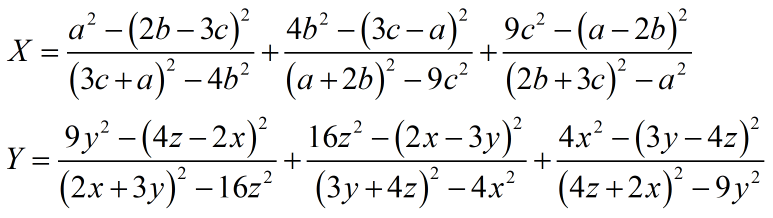Then, find the value of 2017(X + Y).

NMTC Final Stage Previous Year Question For Class 7 and 8: Ques No 4

The sum of the ages of a man and his wife is six times the sum of the ages of their children. Two years ago the sum of their ages was ten times the sum of the ages of their children. Six year hence the sum of their ages will be three times the sum of the ages of their children. How many children do they have?

NMTC Final Stage Previous Year Question For Class 7 and 8: Ques No 5

(a) a, b, c are three natural numbers such that abc = 27846. If a/6 = b + 4 = c – 4. Find the value of a + b + c.

(b) ABCDEFGH is regular octagon with side length equal to a. Find the area of the trapezium ABDG.

NMTC Final Stage Previous Year Question For Class 7 and 8: Ques No 6

(a) If a, b, c are positive real number such that no two of them are equal, show that a(a – b)(a – c) + b(b – c)(b – a) + c(c – a)(c – b) is always positive.

(b) In the figure below, P, Q, R, S are point on the sides of the triangle ABC such that CP = PQ = QB = BA = AR = RS = SC.error: Content is protected !!